# Pool

If water flows into the pool by two inlets, fill the whole for 8 hours. The first inlet filled pool 6 hour longer than second. How long pool take to fill with two inlets separately?

Result

t1 =  19.54 h
t2 =  13.54 h

#### Solution:Checkout calculation with our calculator of quadratic equations.Leave us a comment of example and its solution (i.e. if it is still somewhat unclear...):

Showing 3 comments:Math student
1/t1+1/(t1-10)=1/18
multiply each term by18(t1)(t1-10)
that results in
18(t1-10)+18t1=t1(t1)(t1)-10t1
using the quadratic formula results in t1=-49.6 and 3.63
ubless i made a mistake, your calculations need reexamination!!!        Correct me, please.

6 months ago  2 LikesDr Math
right side of equation is wrong - should be t1*(t1-10) = t12 - 10*t1 now t13-10t1Math student
the problems seems to have changed - - - t2 is now equal t1-6

therefore 1/t1+1/(t1-6)=1/18
multiplying each term by18(t1)(t1-6) ==== 18(t1-6)+18t1=t1(t1-6), simplifying further 18t1-108+18t1=t12-6t1
or 0=t12-6t1-18t1+108
graphing y=18(t1-6)+18t1-t1(t1-6) results in t1=39.25 hours and t2=39.25-6=33.25 hours (same as your NEW answer!!!!#### To solve this verbal math problem are needed these knowledge from mathematics:

Looking for help with calculating harmonic mean? Looking for a statistical calculator? Looking for help with calculating roots of a quadratic equation? Do you have a linear equation or system of equations and looking for its solution? Or do you have quadratic equation? Tip: Our volume units converter will help you with converion of volume units.

## Next similar examples:

1. Trucks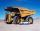Truck take you the some amount of clay in 30 hours. Second truck in 40 hours. Trucks carted the clay away some time together then the second truck broke down and the first one completed the job in five hours. What time worked together?
2. Three workshopsThere are 2743 people working in three workshops. In the second workshop works 140 people more than in the first and in third works 4.2 times more than the second one. How many people work in each workshop?
3. Difference of two numberThe difference of two numbers is 20. They are positive integers greater than zero. The first number raised to one-half equals the second number. Determine the two numbers.
4. Journey to school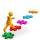On the way to school I went steady step. In the first half of the journey, I counted every second step, in the second half every third. How many steps I do when I went to school if I counted double step are 250 more than three steps?
5. Mom and daughterMother is 39 years old. Her daughter is 15 years. For many years will mother be four times older than the daughter?
6. Elimination methodSolve system of linear equations by elimination method: 5/2x + 3/5y= 4/15 1/2x + 2/5y= 2/15
7. Powers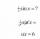Is true for any number a,b,c equality:? ?
8. ProductThe product of two consecutive odd numbers is 8463. What are this numbers?
9. Jane and Miro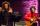Jane brother Miro is 42 years. And he is three times old as it was Jane when Miro was for so many years as there are now Jane. How old is Jane?
10. Threesome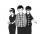Dana, Dalibor and Michael have a combined 57 years. Dana is five years older than Dalibor, but Dana is five years younger than Michael. Determine how old is Dana, Dalibor and Michael.
11. DiscriminantDetermine the discriminant of the equation: ?
12. Reciprocal equationDetermine the root of the equation: 9/x-7/x=1
13. Mother and daughterThe mother is four times older than her daughter. Five years ago, her daughter was seven times younger than her mother. How many years do they have now?
14. Binomials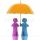To binomial ? add a number to the resulting trinomial be square of binomial.
15. PoojaPooja and Deepa age is 4:5, 4 years back it was 8:11. What is the age of Pooja now?
16. Three figures - numbersThe sum of three numbers, if each is 10% larger than the previous one, is 662. Determine the figures.
17. 13 ticketsA short and long sightseeing tour is possible at the castle. Ticket for a short sightseeing circuit costs CZK 60, for a long touring circuit costs CZK 100. So far, 13 tickets have been sold for 1140 CZK. How much did you pay for tickets for a short tour?